Games
Problems
Go Pro!

# Mathematics

Reference > Mathematics

# Featured Reference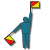Educational Tutorial and code puzzles: Codes, Decoding and Secret Messages: How codes are used, and different types of codes, including morse code, co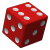Probability lessons - explanation, examples, and word problems involving probability for high school students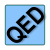What is a proof? Exploration of various types of proofs and how they are usedHow to simplify various kinds of radical expressions, including square roots, cube roots, and expressions with radicals in the denominators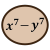Learn more about polynomials and polynomial equations. How to find solutions, understanding conjugates, relation between roots and coefficients, and t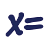Solving systems of equations, including systems of two and three unknowns, as well as fractional equations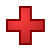A unit on rational expressions, including adding, subtracting, multiplying, dividing algebraic fractions, as well as solving fractional equations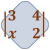A brief unit on calculation, reduction, and uses of determinants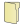# Full Directory Listing

Codes and Secret Messages, Estimation, Introduction to Proofs, Probability, Slick Math, The Golden Ratio, TI-30X II

# Blogs on This SiteReviews and book lists - books we love!The site administrator fields questions from visitors.Like us on Facebook to get updates about new resources